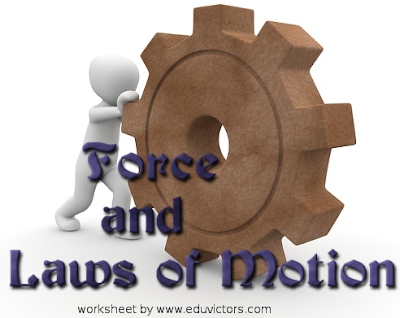## Force and Laws of Motion (Worksheet)

Q: Fill in the blanks with suitable choice given in brackets.

1: The product of mass and velocity of a body is called __________. (momentum/force)

2: The property by the virtue of which an object tends to remain in the state of rest or of uniform motion unless acted upon by some force is called __________. (impulse/ inertia)

3: The SI unit of _________ is kilogram meters per second i.e. kg m/s (momentum/ force)

4: Momentum is a _________ (scalar/ vector) quantity and is directed along the __________ (direction/ magnitude) of velocity.

5: The total momentum of the bullet and the gun before firing would be _________ (zero/ positive / negative) because both of them ar at rest.

6:  ______ forces cannot produce motion in a body but can its shape. (Balanced / Unbalanced)

7: ______ force slows down a moving bicycle when we stop pedaling it. (Frictional / Gravitational)

8:  When an object is under free fall, the Force of gravity acting on the object is a ________ force. (balanced / unbalanced)

9: _________ is the property of bodies to resist a change in their state of rest or motion. (Inertia/ Mass)

10: Newton’s _________ of motion is also known as Galileo’s law of inertia. (Ist law/ IInd Law / IIIrd Law)

11: Force is a _____ quantity. (vector/ scalar)

12: ________ always opposes motion of objects. (Force of friction/ Force of gravity)

13: Action and reaction forces act on _________ but they are equal in magnitude. (two different bodies/ same body)

14: The velocity with which gun moves in the backward direction is known as the ______ velocity. (recoil, initial )

15: Inertia of a body depends on its ________. (mass / velocity)

1: momentum
2: inertia
3: momentum
4: vector, direction
5: zero
6: Balanced
7: Frictional
8: unbalanced
9: Inertia
10: Ist law
11: vector
12: Force of friction
13: two different bodies
14: recoil
15: mass1.2.